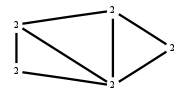# The Markov Bases Database

## G47g_bin

The binary graphical model of G47.

It is a hierarchical model of 5 variables. The dimension of the model is 15.The cardinality of the statespace is 32.

### Properties of the Markov basis

 Markov degree 2 40

 degree 2 40

The model has the following properties:

• All variables are binary.
• It is a graphical model.
• The semigroup is normal.

The following properties of the model are unknown:

• The minimal Markov basis may be unique.### FILES

 Markov basis: sufficient statistics matrix: G47g_bin.mar (3.79 kb) G47g_bin.mat (1.53 kb) G47g_bin.mod (40 b) G47g_bin.tar.gz (8.78 kb)